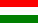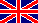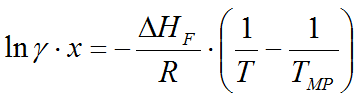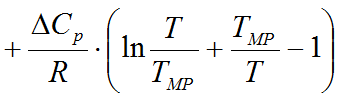solubility.info Calculate solubility in water, ethyl alcohol and other organic solventsQ:   What is Solubility.info for?
A:   Solubility.info is a free application providing solubility data. It calculates (predicts) the solubility of a single compound in a pure organic solvent or in solvent mixtures, using thermodynamic calculations. The results are obtained in a printable report.

Q:   Is this program free of charge?
A:   Yes, the use of Solubility.info is completely free of charge.

Q:   What sort of thermodynamic calculations are used?
A:   The program uses the so-called solubility equation to determine the equilibral concentration in the saturated solution:where
•(gamma) is the activity coefficient of the solute in the saturated solution
• x is the equilibral concentration in the saturated solution (mole fraction)
•HF is the molar fusion ethalpy of the solute (J/mol)
• R is the universal gas constant (8.314 J/mol*K)
• T is the actual temperature of the saturated solution (Kelvin)
• TMP is the melting temperature of the solid substance (Kelvin)
•Cp is the difference of the solute's molar specific heat in the solid and liquid phases (J/mol*K)
The activity coefficients are obtained by the use of the UNIQUAC model and the Statistical Associating Fluid Theory (S.A.F.T.). The required model parameters as well as the necessary physical constants of the compounds are stored in a tiny, compact database.

Q:   What about the reliability of the diagrams/calculation results?
A:   The precision of the results are generally useful for less demanding industrial calculations and educational/demonstrational use. Not recommended for precise calculations and for research.

Q:   Is the program capable to predict mutual solubilities of non-miscible liquids or solubilities of gases?
A:   Not yet, but it is planned.

Q:   Found an error/mistake.
A:   However during building the database and the program the point was to achive maximum reliability, errors can still occur. This service is under continuous development and sorry for any incidental error, but I can not take any responsibility for any damages or losses originating from them. Use the program at your own risk! Corrections are welcome at solubility.info@gmail.com.